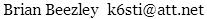# Relating dBµ and dBf

Broadcasters normally specify electromagnetic field strength in dBµ, which is dB above 1 µV/m. Receiver manufacturers typically specify sensitivity in dBf, which is dB above 1 femtowatt (10−15 W). I wondered how the two were related. Originally I used an antenna analysis program to find out. But it's more straightforward to directly use electromagnetics.

The following equation gives the power an antenna captures from an incident electromagnetic wave:

P = E²λ²g / 4πη

where P is power in watts, E is the electric field intensity in volts/meter, λ is wavelength in meters, g is isotropic antenna gain, and η is the impedance of free space.

Substituting numerical constants and converting to dB yields

dBf = dBµ + 10log(λ²g) − 6.76

At 98 MHz, λ = 3.06. For a halfwave dipole, g = 1.64. Therefore, at the middle of the FM broadcast band

dBf = dBµ + 5.1 + G

where G is antenna gain in dBd. G includes conductor, dielectric, mismatch, balun, and feedline losses. The expression is accurate to within 1 dB over 88–108 MHz.

November 30, 201488–108 MHz